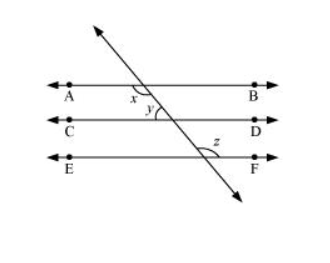# In the given figure, if $A B\|C D, C D\| E F$ and $y: z=3: 7$, find $x$.Solution:

It is given that $A B \| C D$ and $C D \| E F$

$\therefore \mathrm{AB}\|\mathrm{CD}\| \mathrm{EF}$ (Lines parallel to the same line are parallel to each other)

It can be observed that

$x=z$ (Alternate interior angles) ...(1)

It is given that $y: z=3: 7$

Let the common ratio between $y$ and $z$ be $a$.

$\therefore y=3 a$ and $z=7 a$

Also, $x+y=180^{\circ}$ (Co-interior angles on the same side of the transversal)

$z+y=180^{\circ}[U$ sing equation (1) $]$

$7 a+3 a=180^{\circ}$

$10 a=180^{\circ}$

$a=18^{\circ}$

$\therefore x=7 a=7 \times 18^{\circ}=126^{\circ}$